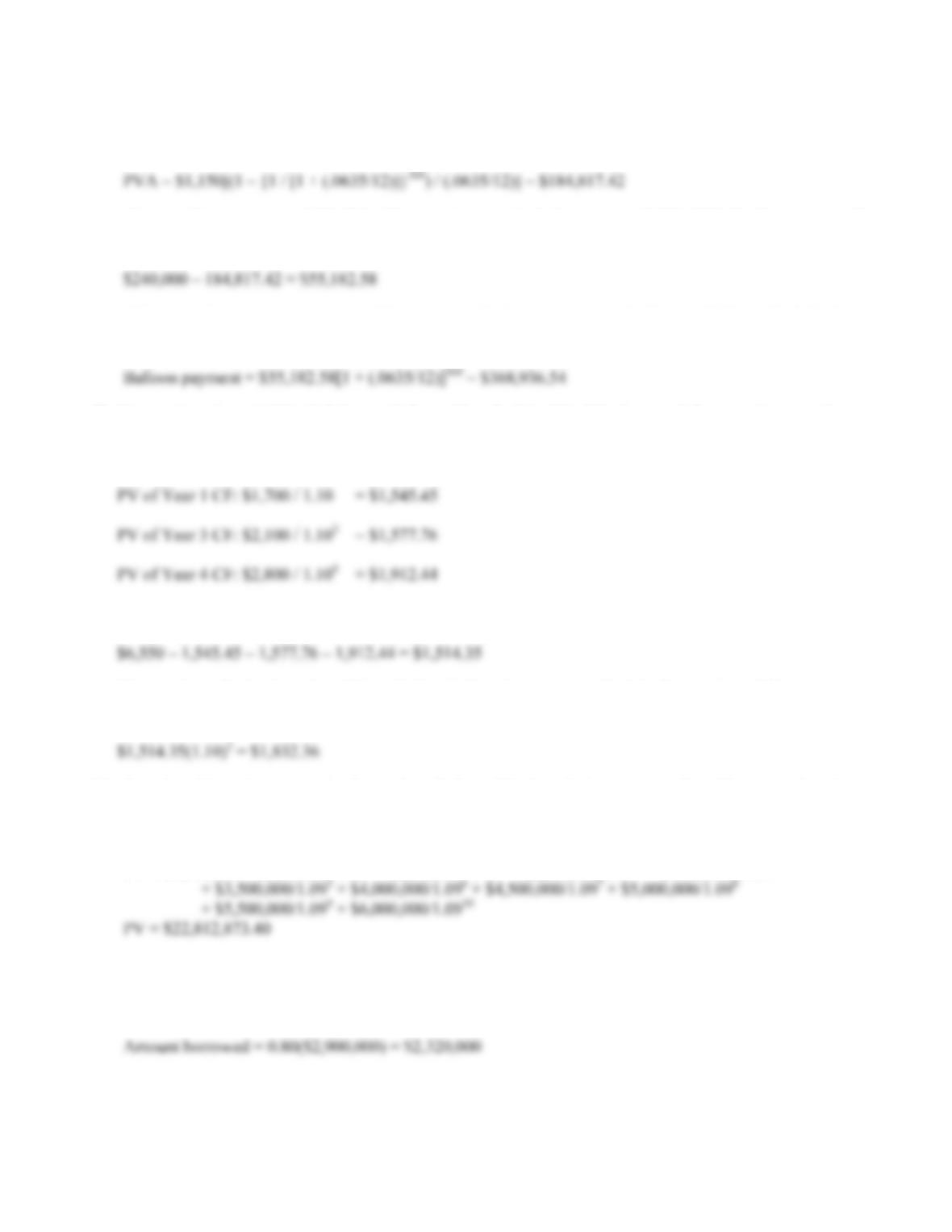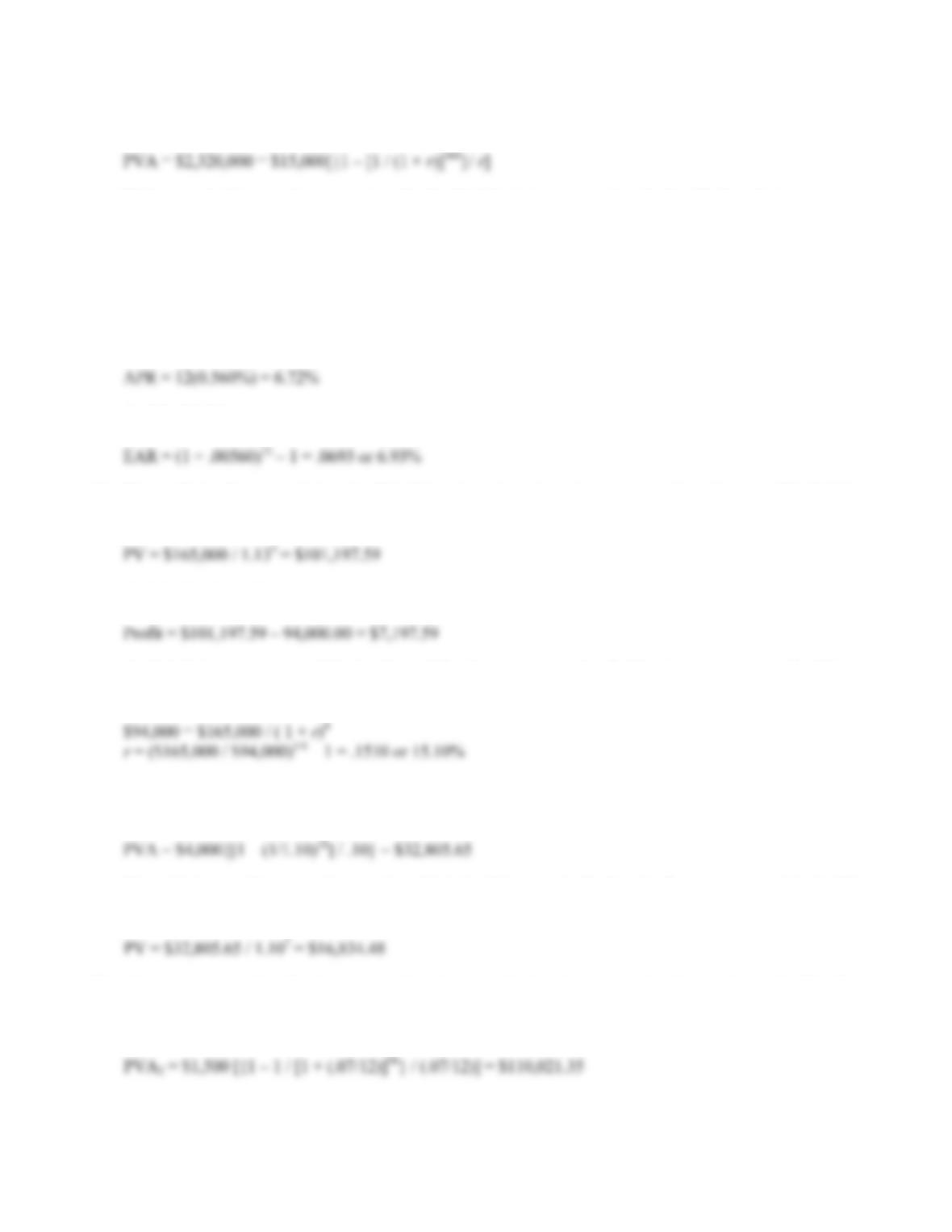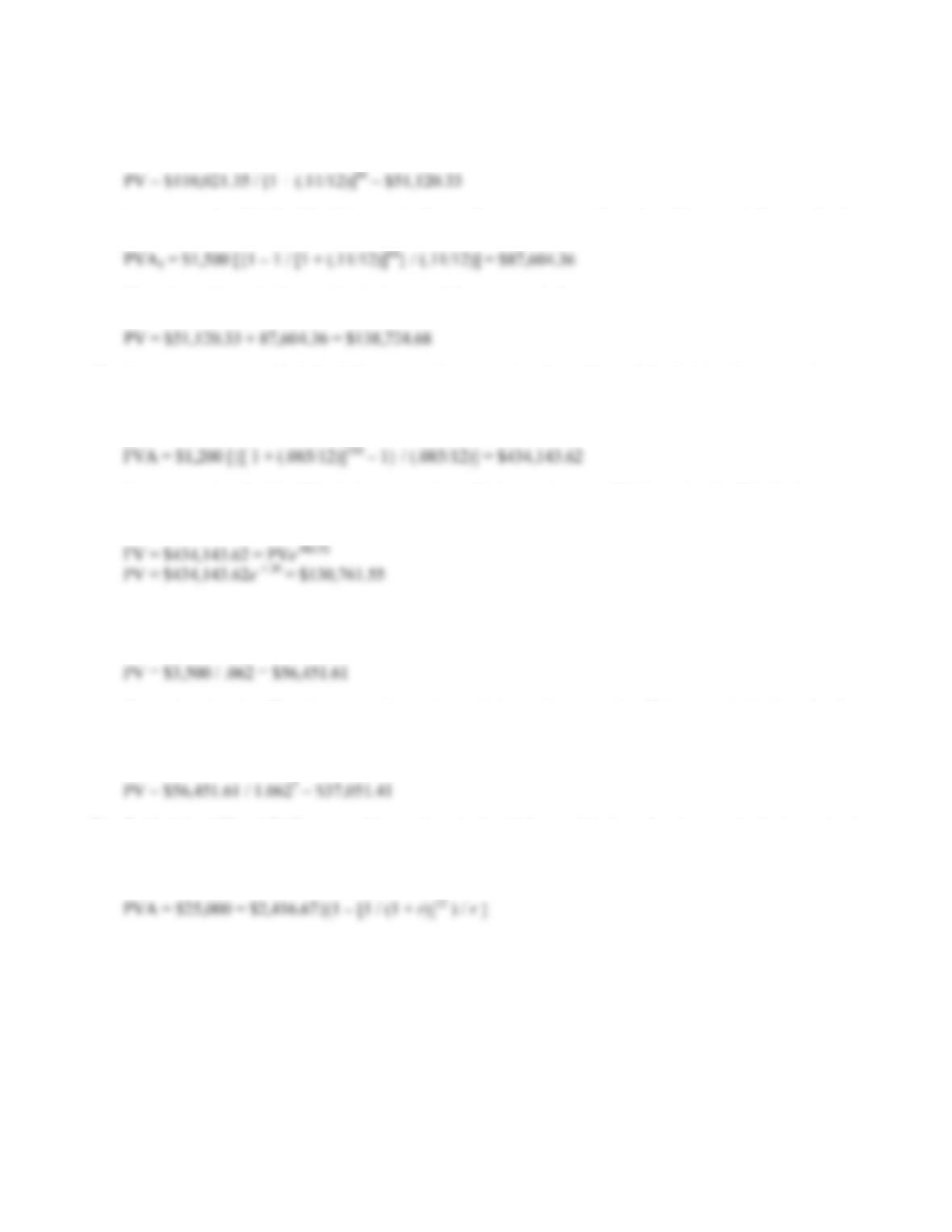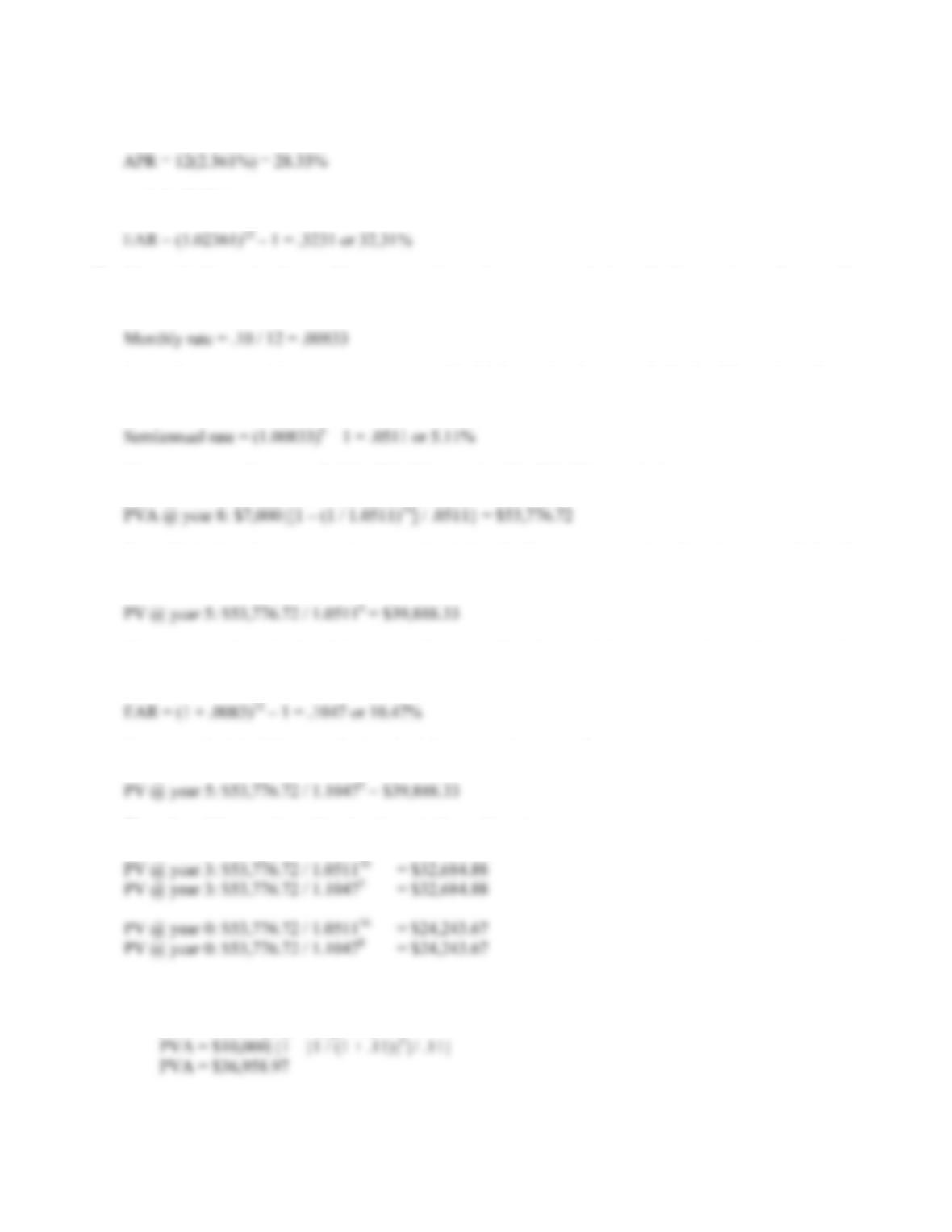# 978-0073382395 Chapter 6 Questions and Problems 42-52

Document Type
Homework Help
Book Title
Fundamentals of Corporate Finance Standard Edition 9th Edition
Authors
Stephen RossCHAPTER 6 B-81
42. The amount of principal paid on the loan is the PV of the monthly payments you make. So, the present
value of the \$1,150 monthly payments is:
The monthly payments of \$1,150 will amount to a principal payment of \$184,817.42. The amount of
principal you will still owe is:
This remaining principal amount will increase at the interest rate on the loan until the end of the loan
period. So the balloon payment in 30 years, which is the FV of the remaining principal will be:
43. We are given the total PV of all four cash flows. If we find the PV of the three cash flows we know, and
subtract them from the total PV, the amount left over must be the PV of the missing cash flow. So, the PV of
the cash flows we know are:
So, the PV of the missing CF is:
The question asks for the value of the cash flow in Year 2, so we must find the future value of this amount.
The value of the missing CF is:
44. To solve this problem, we simply need to find the PV of each lump sum and add them together. It is
important to note that the first cash flow of \$1 million occurs today, so we do not need to discount that cash
flow. The PV of the lottery winnings is:
PV = \$1,000,000 + \$1,500,000/1.09 + \$2,000,000/1.092 + \$2,500,000/1.093 + \$3,000,000/1.094
45. Here we are finding interest rate for an annuity cash flow. We are given the PVA, number of periods, and
the amount of the annuity. We should also note that the PV of the annuity is not the amount borrowed since
we are making a down payment on the warehouse. The amount borrowed is:B-82 SOLUTIONS
Using the PVA equation:
Unfortunately this equation cannot be solved to find the interest rate using algebra. To find the interest rate,
we need to solve this equation on a financial calculator, using a spreadsheet, or by trial and error. If you use
trial and error, remember that increasing the interest rate lowers the PVA, and decreasing the interest rate
increases the PVA. Using a spreadsheet, we find:
r = 0.560%
The APR is the monthly interest rate times the number of months in the year, so:
And the EAR is:
46. The profit the firm earns is just the PV of the sales price minus the cost to produce the asset. We find the
PV of the sales price as the PV of a lump sum:
And the firm’s profit is:
To find the interest rate at which the firm will break even, we need to find the interest rate using the PV (or
FV) of a lump sum. Using the PV equation for a lump sum, we get:
47. We want to find the value of the cash flows today, so we will find the PV of the annuity, and then bring the
lump sum PV back to today. The annuity has 18 payments, so the PV of the annuity is:
Since this is an ordinary annuity equation, this is the PV one period before the first payment, so it is the PV
at t = 7. To find the value today, we find the PV of this lump sum. The value today is:
48. This question is asking for the present value of an annuity, but the interest rate changes during the life of
the annuity. We need to find the present value of the cash flows for the last eight years first. The PV of
these cash flows is:CHAPTER 6 B-83
Note that this is the PV of this annuity exactly seven years from today. Now we can discount this lump sum
to today. The value of this cash flow today is:
Now we need to find the PV of the annuity for the first seven years. The value of these cash flows today is:
The value of the cash flows today is the sum of these two cash flows, so:
49. Here we are trying to find the dollar amount invested today that will equal the FVA with a known interest
rate, and payments. First we need to determine how much we would have in the annuity account. Finding
the FV of the annuity, we get:
Now we need to find the PV of a lump sum that will give us the same FV. So, using the FV of a lump sum
with continuous compounding, we get:
50. To find the value of the perpetuity at t = 7, we first need to use the PV of a perpetuity equation. Using this
equation we find:
Remember that the PV of a perpetuity (and annuity) equations give the PV one period before the first
payment, so, this is the value of the perpetuity at t = 14. To find the value at t = 7, we find the PV of this
lump sum as:
51. To find the APR and EAR, we need to use the actual cash flows of the loan. In other words, the interest rate
quoted in the problem is only relevant to determine the total interest under the terms given. The interest rate
for the cash flows of the loan is:
Again, we cannot solve this equation for r, so we need to solve this equation on a financial calculator, using
a spreadsheet, or by trial and error. Using a spreadsheet, we find:
r = 2.361% per monthB-84 SOLUTIONS
So the APR is:
And the EAR is:
52. The cash flows in this problem are semiannual, so we need the effective semiannual rate. The
interest rate given is the APR, so the monthly interest rate is:
To get the semiannual interest rate, we can use the EAR equation, but instead of using 12 months as the
exponent, we will use 6 months. The effective semiannual rate is:
We can now use this rate to find the PV of the annuity. The PV of the annuity is:
Note, this is the value one period (six months) before the first payment, so it is the value at year 8. So, the
value at the various times the questions asked for uses this value 8 years from now.
Note, you can also calculate this present value (as well as the remaining present values) using the number
of years. To do this, you need the EAR. The EAR is:
So, we can find the PV at year 5 using the following method as well:
The value of the annuity at the other times in the problem is:
53. a. If the payments are in the form of an ordinary annuity, the present value will be:
PVA = C({1 – [1/(1 + r)t]} / r ))

## Trusted by Thousands ofStudents

Here are what students say about us.